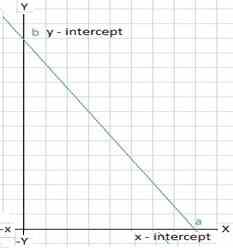# Two Intercept form Calculator

Calculator calculate the equation of a straight line by putting the values for x and y co-ordinates.

 x - intercept(a): y - intercept(b):

 Equation :

Calculated Equation of a straight line
using following formula :
$$\frac{x}{a} + \frac{y}{b} = 1$$

A line passing through two points,this is used to generate the equation of a straight line with x - intercept a and y - intercept b of the line.### Examples

Let's take the example of straight line intercepting x-axis at 8 and intercepting y-axis at 14,
so we can say a=8 and y=14.
Now we can apply the values in formula: $$\frac{x}{a} + \frac{y}{b} = 1$$ $$\frac{x}{8} + \frac{y}{14} = 1$$ $$\frac{14x + 8y}{112} = 1$$ $$14x + 8y = 112$$